# Tag: Connes

This time of year I’m usually in France, or at least I was before Covid. This might explain for my recent obsession with French math YouTube interviews.

Today’s first one is about Bourbaki’s golden years, the period between WW2 and 1975. Alain Connes is trying to get some anecdotes from Jean-Pierre Serre, Pierre Cartier, and Jacques Dixmier.

If you don’t have the time to sit through the whole thing, perhaps you might have a look at the discussion on whether or not to include categories in Bourbaki (starting at 51.40 into the clip).

Here are some other time-slots (typed on a qwerty keyboard, mes excuses) with some links.

• 8.59 : Canular stupide (mort de Bourbaki)
• 15.45 : recrutement de Koszul
• 17.45 : recrutement de Grothendieck
• 26.15 : influence de Serre
• 28.05 : importance des ultra filtres
• 35.35 : Meyer
• 37.20 : faisceaux
• 51.00 : Grothendieck
• 51.40 : des categories, Gabriel-Demazure
• 57.50 : lemme de Serre, theoreme de Weil
• 1.03.20 : Chevalley vs. Godement
• 1.05.26 : retraite Dieudonne
• 1.07.05 : retraite
• 1.10.00 : Weil vs. Serre-Borel
• 1.13.50 : hierarchie Bourbaki
• 1.20.22 : categories
• 1.21.30 : Bourbaki, une secte?
• 1.22.15 : Grothendieck C.N.R.S. 1984

The second one is an interview conducted by Alain Connes with Jean-Pierre Serre on the Grothendieck-Serre correspondence.

Again, if you don’t have the energy to sit through it all, perhaps I can tempt you with Serre’s reaction to Connes bringing up the subject of toposes (starting at 14.36 into the clip).

• 2.10 : 2e these de Grothendieck: des faisceaux
• 3.50 : Grothendieck -> Bourbaki
• 6.46 : Tohoku
• 8.00 : categorie des diagrammes
• 9.10 : schemas et Krull
• 10.50 : motifs
• 11.50 : cohomologie etale
• 14.05 : Weil
• 14.36 : topos
• 16.30 : Langlands
• 19.40 : Grothendieck, cours d’ecologie
• 24.20 : Dwork
• 25.45 : Riemann-Roch
• 29.30 : influence de Serre
• 30.50 : fin de correspondence
• 32.05 : pourquoi?
• 33.10 : SGA 5
• 34.50 : methode G. vs. theorie des nombres
• 37.00 : paranoia
• 37.15 : Grothendieck = centrale nucleaire
• 38.30 : Clef des songes
• 42.35 : 30.000 pages, probleme du mal
• 44.25 : Ribenboim
• 45.20 : Grothendieck a Paris, publication R et S
• 48.00 : 50 ans IHES, lettre a Bourguignon
• 50.46 : Laurant Laforgue
• 51.35 : Lasserre
• 53.10 : l’humour

“She simply walked into the pond in Kensington Gardens Sunday morning and drowned herself in three feet of water.”

This is the opening sentence of The Ishango Bone, a novel by Paul Hastings Wilson. It (re)tells the story of a young mathematician at Cambridge, Amiele, who (dis)proves the Riemann Hypothesis at the age of 26, is denied the Fields medal, and commits suicide.

In his review of the novel on MathFiction, Alex Kasman casts he story in the 1970ties, based on the admission of the first female students to Trinity.

More likely, the correct time frame is in the first decade of this century. On page 121 Amiele meets Alain Connes, said to be a “past winner of the Crafoord Prize”, which Alain obtained in 2001. In fact, noncommutative geometry and its interaction with quantum physics plays a crucial role in her ‘proof’.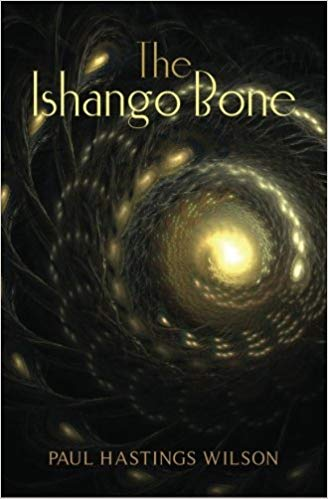The Ishango artefact only appears in the Coda to the book. There are a number of theories on the nature and grouping of the scorings on the bone. In one column some people recognise the numbers 11, 13, 17 and 19 (the primes between 10 and 20).

In the book, Amiele remarks that the total number of lines scored on the bone (168) “happened to be the exact total of all the primes between 1 and 1000” and “if she multiplied 60, the total number of lines in one side column, by 168, the grand total of lines, she’d get 10080,…,not such a far guess from 9592, the actual total of primes between 1 and 100000.” (page 139-140)

The bone is believed to be more than 20000 years old, prime numbers were probably not understood until about 500 BC…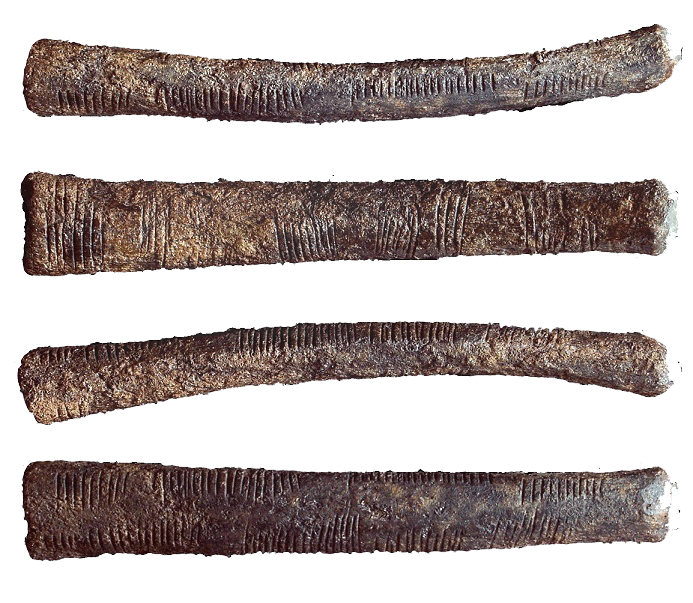More interesting than these speculations on the nature of the Ishango bone is the description of the tools Amiele thinks to need to tackle the Riemann Hypothesis:

“These included algebraic geometry (which combines commutative algebra with the language and problems of geometry); noncommutative geometry (concerned with the geometric approach to associative algebras, in which multiplication is not commutative, that is, for which $x$ times $y$ does not always equal $y$ times $x$); quantum field theory on noncommutative spacetime, and mathematical aspects of quantum models of consciousness, to name a few.” (page 115)

The breakthrough came two years later when Amiele was giving a lecture on Grothendieck’s dessins d’enfant.

“Dessin d’enfant, or ‘child’s drawing’, which Amiele had discovered in Grothendieck’s work, is a type of graph drawing that seemed technically simple, but had a very strong impression on her, partly due to the familiar nature of the objects considered. (…) Amiele found subtle arithmetic invariants associated with these dessins, which were completely transformed, again, as soon as another stroke was added.” (page 116)

Amiele’s ‘disproof’ of RH is outlined on pages 122-124 of “The Ishango Bone” and is a mixture of recognisable concepts and ill-defined terms.

“Her final result proved that Riemann’s Hypothesis was false, a zero must fall to the east of Riemann’s critical line whenever the zeta function of point $q$ with momentum $p$ approached the aelotropic state-vector (this is a simplification, of course).” (page 123)

More details are given in a footnote:

“(…) a zero must fall to the east of Riemann’s critical line whenever:

$\zeta(q_p) = \frac{( | \uparrow \rangle + \Psi) + \frac{1}{2}(1+cos(\Theta))\frac{\hbar}{\pi}}{\int(\Delta_p)}$

(…) The intrepid are invited to try the equation for themselves.” (page 124)

Wilson’s “The Ishango Bone” was published in 2012. A fair number of topics covered (the Ishango bone, dessin d’enfant, Riemann hypothesis, quantum theory) also play a prominent role in the 2015 paper/story by Michel Planat “A moonshine dialogue in mathematical physics”, but this time with additional story-line: monstrous moonshine

Such a paper surely deserves a separate post.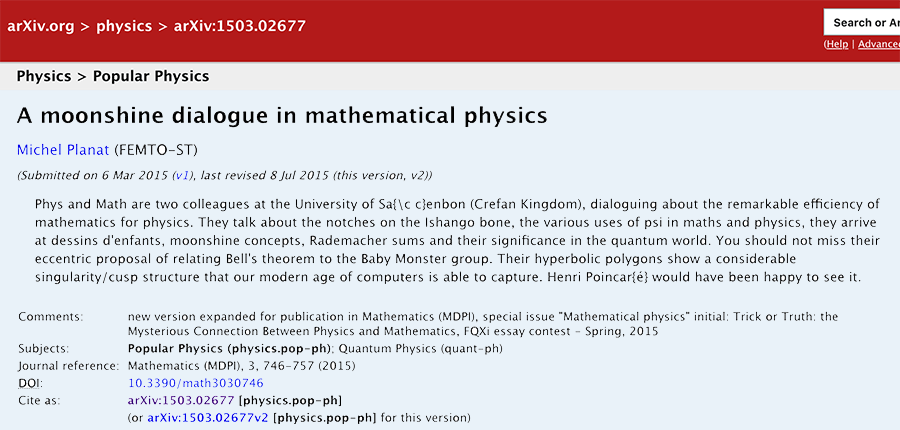‘Gabriel’s topos’ (see here) is the conjectural, but still elusive topos from which the validity of the Riemann hypothesis would follow.

It is the latest attempt in Alain Connes’ 20 year long quest to tackle the RH (before, he tried the tools of noncommutative geometry and later those offered by the field with one element).

For the last 5 years he hopes that topos theory might provide the missing ingredient. Together with Katia Consani he introduced and studied the geometry of the Arithmetic site, and later the geometry of the scaling site.

If you look at the points of these toposes you get horribly complicated ‘non-commutative’ spaces, such as the finite adele classes $\mathbb{Q}^*_+ \backslash \mathbb{A}^f_{\mathbb{Q}} / \widehat{\mathbb{Z}}^{\ast}$ (in case of the arithmetic site) and the full adele classes $\mathbb{Q}^*_+ \backslash \mathbb{A}_{\mathbb{Q}} / \widehat{\mathbb{Z}}^{\ast}$ (for the scaling site).

In Vienna, Connes gave a nice introduction to the arithmetic site in two lectures. The first part of the talk below also gives an historic overview of his work on the RH

The second lecture can be watched here.

However, not everyone is as optimistic about the topos-approach as he seems to be. Here’s an insightful answer on MathOverflow by Will Sawin to the question “What is precisely still missing in Connes’ approach to RH?”.

Other interesting MathOverflow threads related to the RH-approach via the field with one element are Approaches to Riemann hypothesis using methods outside number theory and Riemann hypothesis via absolute geometry.

About a month ago, from May 10th till 14th Alain Connes gave a series of lectures at Ohio State University with title “The Riemann-Roch strategy, quantizing the Scaling Site”.

The accompanying paper has now been arXived: The Riemann-Roch strategy, Complex lift of the Scaling Site (joint with K. Consani).

Especially interesting is section 2 “The geometry behind the zeros of $\zeta$” in which they explain how looking at the zeros locus inevitably leads to the space of adele classes and why one has to study this space with the tools from noncommutative geometry.

Perhaps further developments will be disclosed in a few weeks time when Connes is one of the speakers at Toposes in Como.No kidding, this is the final sentence of Le spectre d’Atacama, the second novel by Alain Connes (written with Danye Chéreau (IRL Mrs. AC) and his former Ph.D. advisor Jacques Dixmier).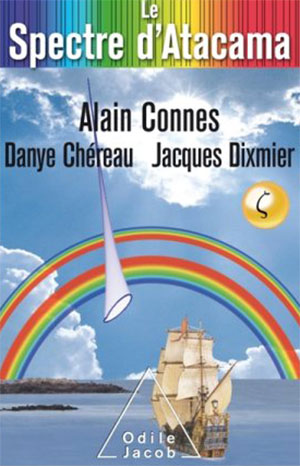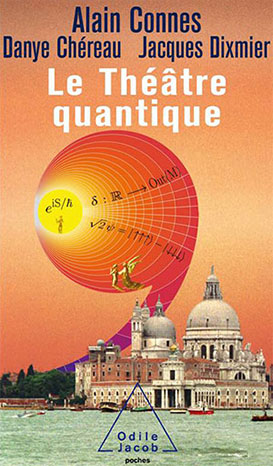The book has a promising start. Armand Lafforet (IRL AC) is summoned by his friend Rodrigo to the Chilean observatory Alma in the Altacama desert. They have observed a mysterious spectrum, and need his advice.

Armand drops everything and on the flight he lectures the lady sitting next to him on proofs by induction (breaking up chocolate bars), and recalls a recent stay at the La Trappe Abbey, where he had an encounter with (the ghost of) Alexander Grothendieck, who urged him to ‘Follow the motif!’.

“Comment était-il arrivé là? Il possédait surement quelques clés. Pourquoi pas celles des songes?” (How did he get
there? Surely he owned some keys, why not those of our dreams?)

A few pages further there’s this on the notion of topos (my attempt to translate):

“The notion of space plays a central role in mathematics. Traditionally we represent it as a set of points, together with a notion of neighborhood that we call a ‘topology’. The universe of these new spaces, ‘toposes’, unveiled by Grothendieck, is marvellous, not only for the infinite wealth of examples (it contains, apart from the ordinary topological spaces, also numerous instances of a more combinatorial nature) but because of the totally original way to perceive space: instead of appearing on the main stage from the start, it hides backstage and manifests itself as a ‘deus ex machina’, introducing a variability in the theory of sets.”

So far, so good.

We have a mystery, tidbits of mathematics, and allusions left there to put a smile on any Grothendieck-aficionado’s face.

But then, upon arrival, the story drops dead.

Rodrigo has been taken to hospital, and will remain incommunicado until well in the final quarter of the book.

As the remaining astronomers show little interest in Alain’s (sorry, Armand’s) first lecture, he decides to skip the second, and departs on a hike to the ocean. There, he takes a genuine sailing ship in true Jules Verne style to the lighthouse at he end of the world.

All this drags on for at least half a year in time, and two thirds of the book’s length. We are left in complete suspense when it comes to the mysterious Atacama spectrum.

Perhaps the three authors deliberately want to break with existing conventions of story telling?

I had a similar feeling when reading their first novel Le Theatre Quantique. Here they spend some effort to flesh out their heroine, Charlotte, in the first part of the book. But then, all of a sudden, their main character is replaced by a detective, and next by a computer.

Anyway, when Armand finally reappears at the IHES the story picks up pace.

The trio (Armand, his would-be-lover Charlotte, and Ali Ravi, Cern’s computer guru) convince CERN to sell its main computer to an American billionaire with the (fake) promise of developing a quantum computer. Incidentally, they somehow manage to do this using Charlotte’s history with that computer (for this, you have to read ‘Le Theatre Quantique’).

By their quantum-computing power (Shor and quantum-encryption pass the revue) they are able to decipher the Atacame spectrum (something to do with primes and zeroes of the zeta function), send coded messages using quantum entanglement, end up in the Oval Office and convince the president to send a message to the ‘Riemann sphere’ (another fun pun), and so on, and on.

The book ends with a twist of the classic tale of the mathematician willing to sell his soul to the devil for a (dis)proof of the Riemann hypothesis:

After spending some time in purgatory, the mathematician gets a meeting with God and asks her the question “Is the Riemann hypothesis true?”.

“Of course”, God says.

“But how can you know that all non-trivial zeroes of the zeta function have real part 1/2?”, Armand asks.

And God replies:

“Simple enough, I can see them all at once. But then, don’t forget I’m God. I can see the disappointment in your face, yes I can read in your heart that you are frustrated, that you desire an explanation…

Well, we’re going to fix this. I will call archangel Gabriel, the angel of geometry, he will make you a topos!”

If you feel like running to the nearest Kindle store to buy “Le spectre d’Atacama”, make sure to opt for a package deal. It is impossible to make heads or tails of the story without reading “Le theatre quantique” first.

But then, there are worse ways to spend an idle week than by binge reading Connes…

Edit (February 28th). A short video of Alain Connes explaining ‘Le spectre d’Atacama’ (in French)

The Bulletin of the AMS just made this paper by Julia Mueller available online: “On the genesis of Robert P. Langlands’ conjectures and his letter to Andre Weil” (hat tip +ChandanDalawat and +DavidRoberts on Google+).

It recounts the story of the early years of Langlands and the first years of his mathematical career (1960-1966)leading up to his letter to Andre Weil in which he outlines his conjectures, which would become known as the Langlands program.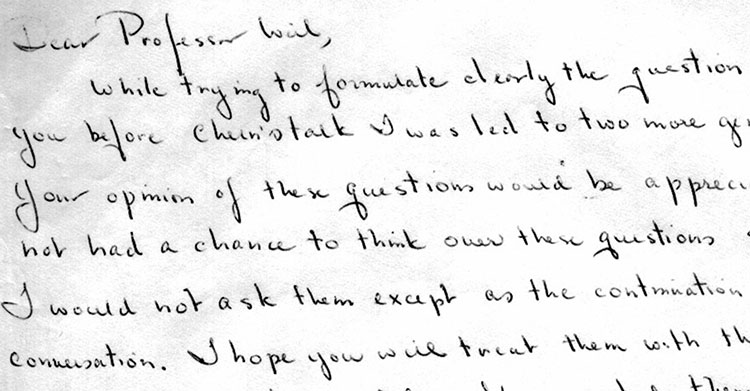Langlands letter to Weil is available from the IAS.

The Langlands program is a vast net of conjectures. For example, it conjectures that there is a correspondence between

– $n$-dimensional representations of the absolute Galois group $Gal(\overline{\mathbb{Q}}/\mathbb{Q})$, and

– specific data coming from an adelic quotient-space $GL_n(\mathbb{A}_{\mathbb{Q}})/GL_n(\mathbb{Q})$.

For $n=1$ this is essentially class field theory with the correspondence given by Artin’s reciprocity law.

Here we have on the one hand the characters of the abelianised absolute Galois group

$Gal(\overline{\mathbb{Q}}/\mathbb{Q})^{ab} \simeq Gal(\mathbb{Q}(\pmb{\mu}_{\infty})/\mathbb{Q}) \simeq \widehat{\mathbb{Z}}^{\ast}$

and on the other hand the connected components of the idele class space

$GL_1(\mathbb{A}_{\mathbb{Q}})/GL_1(\mathbb{Q}) = \mathbb{A}_{\mathbb{Q}}^{\ast} / \mathbb{Q}^{\ast} = \mathbb{R}_+^{\ast} \times \widehat{\mathbb{Z}}^{\ast}$

For $n=2$ it involves the study of Galois representations coming from elliptic curves. A gentle introduction to the general case is Mark Kisin’s paper What is … a Galois representation?.

One way to look at some of the quantum statistical systems studied via non-commutative geometry is that they try to understand the “bad” boundary of the Langlands space $GL_n(\mathbb{A}_{\mathbb{Q}})/GL_n(\mathbb{Q})$.

Here, the Bost-Connes system corresponds to the $n=1$ case, the Connes-Marcolli system to the $n=2$ case.

If $\mathbb{A}’_{\mathbb{Q}}$ is the subset of all adeles having almost all of its terms in $\widehat{\mathbb{Z}}_p^{\ast}$, then there is a well-defined map

$\pi~:~\mathbb{A}’_{\mathbb{Q}}/\mathbb{Q}^{\ast} \rightarrow \mathbb{R}_+ \qquad (x_{\infty},x_2,x_2,\dots) \mapsto | x_{\infty} | \prod_p | x_p |_p$

The inverse image of $\pi$ over $\mathbb{R}_+^{\ast}$ are exactly the idele classes $\mathbb{A}_{\mathbb{Q}}^{\ast}/\mathbb{Q}^{\ast}$, so we can view them as the nice locus of the horrible complicated quotient of adele-classes $\mathbb{A}_{\mathbb{Q}}/\mathbb{Q}^*$. And we can view the adele-classes as a ‘closure’ of the idele classes.

But, the fiber $\pi^{-1}(0)$ has horrible topological properties because $\mathbb{Q}^*$ acts ergodically on it due to the fact that $log(p)/log(q)$ is irrational for distinct primes $p$ and $q$.

This is why it is better to view the adele-classes not as an ordinary space (one with bad topological properties), but rather as a ‘non-commutative’ space because it is controlled by a non-commutative algebra, the Bost-Connes algebra.

For $n=2$ there’s a similar story with a ‘bad’ quotient $M_2(\mathbb{A}_{\mathbb{Q}})/GL_2(\mathbb{Q})$, being the closure of an ‘open’ nice piece which is the Langlands quotient space $GL_2(\mathbb{A}_{\mathbb{Q}})/GL_2(\mathbb{Q})$.

The monstrous moonshine picture is the finite piece of Conway’s Big Picture needed to understand the 171 moonshine groups associated to conjugacy classes of the monster.

Last time I claimed that there were exactly 7 types of local behaviour, but I missed one. The forgotten type is centered at the number lattice $84$.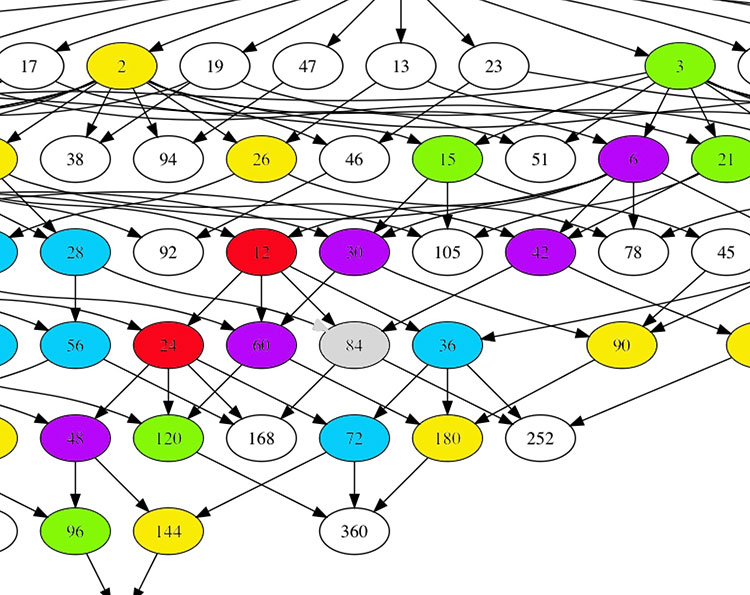Locally around it the moonshine picture looks like this
$\xymatrix{42 \ar@{-}[dr] & 28 \frac{1}{3} \ar@[red]@{-}[d] & 41 \frac{1}{2} \ar@{-}[ld] \\ 28 \ar@[red]@{-}[r] & \color{grey}{84} \ar@[red]@{-}[r] \ar@[red]@{-}[d] \ar@{-}[rd] & 28 \frac{2}{3} \\ & 252 & 168}$

and it involves all square roots of unity ($42$, $42 \frac{1}{2}$ and $168$) and $3$-rd roots of unity ($28$, $28 \frac{1}{3}$, $28 \frac{2}{3}$ and $252$) centered at $84$.

No, I’m not hallucinating, there are indeed $3$ square roots of unity and $4$ third roots of unity as they come in two families, depending on which of the two canonical forms to express a lattice is chosen.

In the ‘normal’ expression $M \frac{g}{h}$ the two square roots are $42$ and $42 \frac{1}{2}$ and the three third roots are $28, 28 \frac{1}{3}$ and $28 \frac{2}{3}$. But in the ‘other’ expression
$M \frac{g}{h} = (\frac{g’}{h},\frac{1}{h^2M})$
(with $g.g’ \equiv 1~mod~h$) the families of $2$-nd and $3$-rd roots of unity are
$\{ 42 \frac{1}{2} = (\frac{1}{2},\frac{1}{168}), 168 = (0,\frac{1}{168}) \}$
and
$\{ 28 \frac{1}{3} = (\frac{1}{3},\frac{1}{252}), 28 \frac{2}{3} = (\frac{2}{3},\frac{1}{252}), 252 = (0 , \frac{1}{252}) \}$
As in the tetrahedral snake post, it is best to view the four $3$-rd roots of unity centered at $84$ as the vertices of a tetrahedron with center of gravity at $84$. Power maps in the first family correspond to rotations along the axis through $252$ and power maps in the second family are rotations along the axis through $28$.

In the ‘normal’ expression of lattices there’s then a total of 8 different local types, but two of them consist of just one number lattice: in $8$ the local picture contains all square, $4$-th and $8$-th roots of unity centered at $8$, and in $84$ the square and $3$-rd roots.

Perhaps surprisingly, if we redo everything in the ‘other’ expression (and use the other families of roots of unity), then the moonshine picture has only 7 types of local behaviour. The forgotten type $84$ appears to split into two occurrences of other types (one with only square roots of unity, and one with only $3$-rd roots).

I wonder what all this has to do with the action of the Bost-Connes algebra on the big picture or with Plazas’ approach to moonshine via non-commutative geometry.

All lattices in the moonshine picture are number-like, that is of the form $M \frac{g}{h}$ with $M$ a positive integer and $0 \leq g < h$ with $(g,h)=1$. To understand the action of the Bost-Connes algebra on the Big Picture it is sometimes better to view the lattice $M \frac{g}{h}$ as a primitive $h$-th root of unity, centered at $hM$.

The distance from $M$ to any of the lattices $M \frac{g}{h}$ is equal to $2 log(h)$, and the distances from $M$ and $M \frac{g}{h}$ to $hM$ are all equal to $log(h)$.

For a prime value $h$, these $h$ lattices are among the $h+1$ lattices branching off at $hM$ in the $h$-adic tree (the remaining one being $h^2M$).

For general $h$ the situation is more complex. Here’s the picture for $h=6$ with edges in the $2$-adic tree painted blue, those in the $3$-adic tree red.

$\xymatrix{& & M \frac{1}{2} \ar@[blue]@{-}[d] & \\ & M \ar@[blue]@{-}[r] \ar@[red]@{-}[d] & 2M \ar@[red]@{-}[d] & M \frac{1}{6} \ar@[red]@{-}[d] \\ M \frac{1}{3} \ar@[red]@{-}[r] & 3M \ar@[blue]@{-}[r] \ar@[red]@{-}[d] & \boxed{6 M} \ar@[blue]@{-}[r] & 3M \frac{1}{2} \ar@[red]@{-}[d] \\ & M \frac{2}{3} & & M \frac{5}{6}}$

To describe the moonshine group $(n|h)+e,f,\dots$ (an example was worked out in the tetrahedral snake post), we need to study the action of base-change with the matrix
$x = \begin{bmatrix} 1 & \frac{1}{h} \\ 0 & 1 \end{bmatrix}$
which sends a lattice of the form $M \frac{g}{h}$ with $0 \leq g < h$ to $M \frac{g+M}{h}$, so is a rotation over $\frac{2 \pi M}{h}$ around $h M$. But, we also have to describe the base-change action with the matrix $y = \begin{bmatrix} 1 & 0 \\ n & 1 \end{bmatrix}$ and for this we better use the second description of the lattice as $M \frac{g}{h}=(\frac{g'}{h},\frac{1}{h^2M})$ with $g'$ the multiplicative inverse of $g$ modulo $h$. Under the action by $y$, the second factor $\frac{1}{h^2M}$ will be fixed, so this time we have to look at all lattices of the form $(\frac{g}{h},\frac{1}{h^2M})$ with $0 \leq g < h$, which again can be considered as another set of $h$-th roots of unity, centered at $hM$. Here's this second interpretation for $h=6$: $\xymatrix{M \frac{5}{6} \ar@[red]@{-}[d] & & 4M \frac{1}{3} \ar@[red]@{-}[d] & \\ 3M \frac{1}{2} \ar@[blue]@{-}[r] \ar@[red]@{-}[d] & \boxed{6M} \ar@[blue]@{-}[r] \ar@[red]@{-}[d] & 12 M \ar@[red]@{-}[r] \ar@[red]@{-}[d] & 4 M \frac{2}{3} \\ M \frac{1}{6} & 18 M \ar@[blue]@{-}[r] \ar@[blue]@{-}[d] & 36 M & \\ & 9M \frac{1}{2} & & }$ Under $x$ the first set of $h$-th roots of unity centered at $hM$ is permuted, whereas $y$ permutes the second set of $h$-th roots of unity.
These interpretations can be used to spot errors in computing the finite groups $\Gamma_0(n|h)/\Gamma_0(n.h)$.

Here’s part of the calculation of the action of $y$ on the $(360|1)$-snake (which consists of $60$-lattices).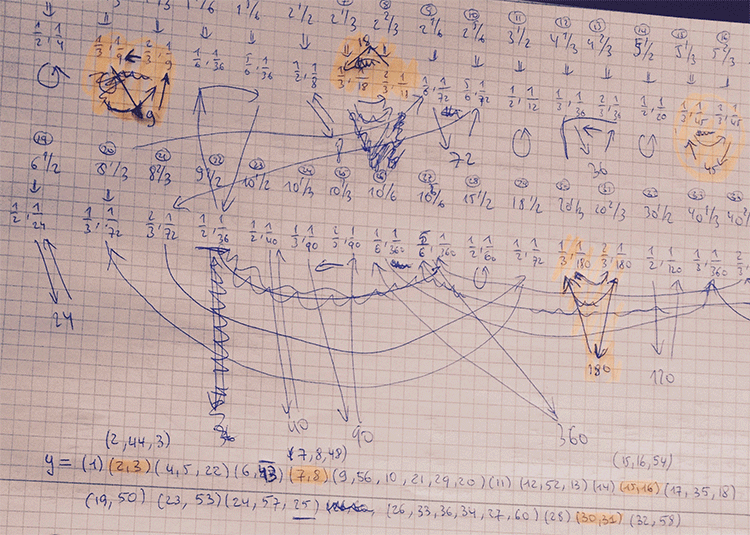First I got a group of order roughly $600.000$. After correcting some erroneous cycles, the order went down to 6912.

Finally I spotted that I mis-numbered two lattices in the description of $x$ and $y$, and the order went down to $48$ as it should, because I knew it had to be equal to $C_2 \times C_2 \times A_4$.

A well-known link between Conway’s Big Picture and non-commutative geometry is given by the Bost-Connes system.

This quantum statistical mechanical system encodes the arithmetic properties of cyclotomic extensions of $\mathbb{Q}$.

The corresponding Bost-Connes algebra encodes the action by the power-maps on the roots of unity.

It has generators $e_n$ and $e_n^*$ for every natural number $n$ and additional generators $e(\frac{g}{h})$ for every element in the additive group $\mathbb{Q}/\mathbb{Z}$ (which is of course isomorphic to the multiplicative group of roots of unity).

The defining equations are
$\begin{cases} e_n.e(\frac{g}{h}).e_n^* = \rho_n(e(\frac{g}{h})) \\ e_n^*.e(\frac{g}{h}) = \Psi^n(e(\frac{g}{h}).e_n^* \\ e(\frac{g}{h}).e_n = e_n.\Psi^n(e(\frac{g}{h})) \\ e_n.e_m=e_{nm} \\ e_n^*.e_m^* = e_{nm}^* \\ e_n.e_m^* = e_m^*.e_n~\quad~\text{if (m,n)=1} \end{cases}$

Here $\Psi^n$ are the power-maps, that is $\Psi^n(e(\frac{g}{h})) = e(\frac{ng}{h}~mod~1)$, and the maps $\rho_n$ are given by
$\rho_n(e(\frac{g}{h})) = \sum e(\frac{i}{j})$
where the sum is taken over all $\frac{i}{j} \in \mathbb{Q}/\mathbb{Z}$ such that $n.\frac{i}{j}=\frac{g}{h}$.

Conway’s Big Picture has as its vertices the (equivalence classes of) lattices $M,\frac{g}{h}$ with $M \in \mathbb{Q}_+$ and $\frac{g}{h} \in \mathbb{Q}/\mathbb{Z}$.

The Bost-Connes algebra acts on the vector-space with basis the vertices of the Big Picture. The action is given by:
$\begin{cases} e_n \ast \frac{c}{d},\frac{g}{h} = \frac{nc}{d},\rho^m(\frac{g}{h})~\quad~\text{with m=(n,d)} \\ e_n^* \ast \frac{c}{d},\frac{g}{h} = (n,c) \times \frac{c}{nd},\Psi^{\frac{n}{m}}(\frac{g}{h})~\quad~\text{with m=(n,c)} \\ e(\frac{a}{b}) \ast \frac{c}{d},\frac{g}{h} = \frac{c}{d},\Psi^c(\frac{a}{b}) \frac{g}{h} \end{cases}$

This connection makes one wonder whether non-commutative geometry can shed a new light on monstrous moonshine?

This question is taken up by Jorge Plazas in his paper Non-commutative geometry of groups like $\Gamma_0(N)$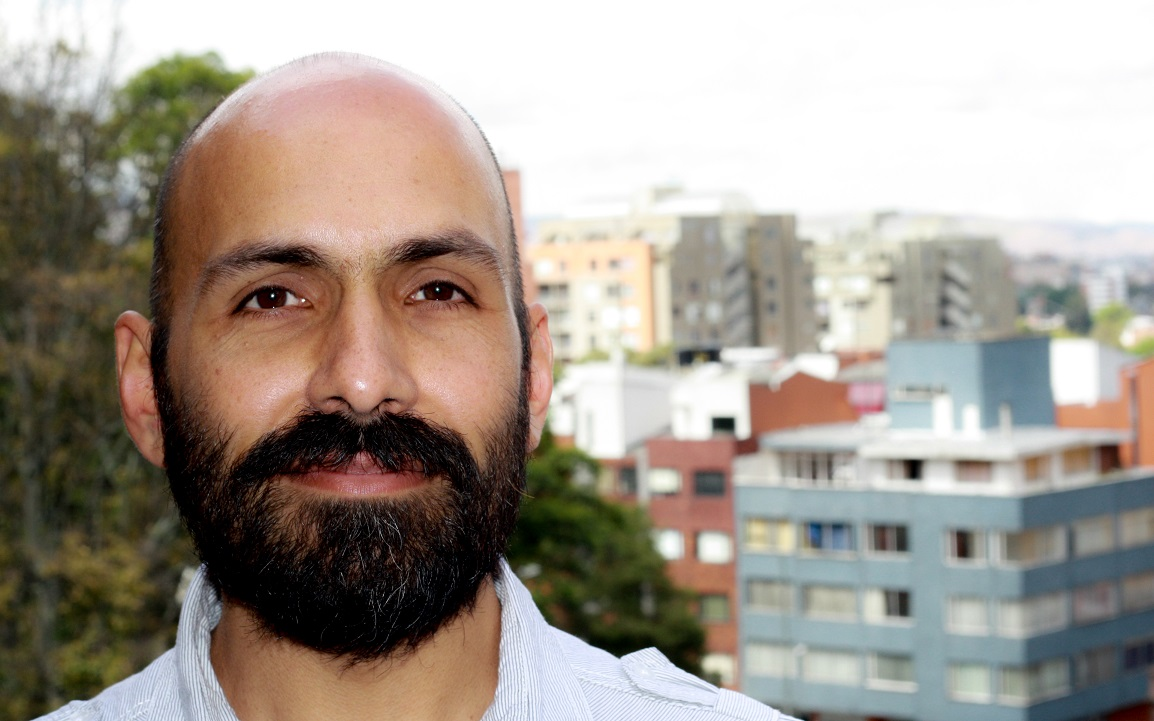Plazas shows that the bigger Connes-Marcolli $GL_2$-system also acts on the Big Picture. An intriguing quote:

“Our interest in the $GL_2$-system comes from the fact that its thermodynamic properties encode the arithmetic theory of modular functions to an extend which makes it possible for us to capture aspects of moonshine theory.”

Looks like the right kind of paper to take along when I disappear next week for some time in the French mountains…